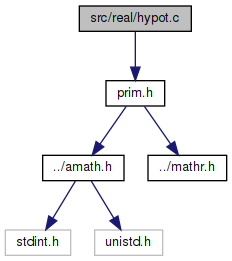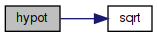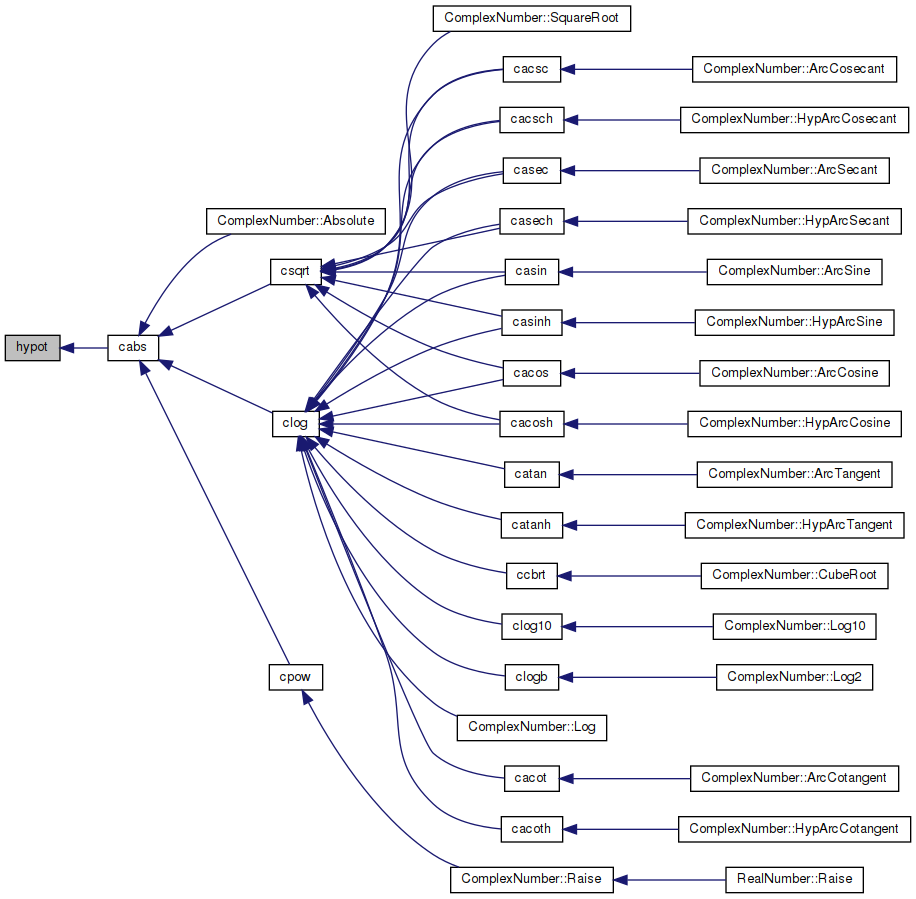amath  1.8.5 Simple command line calculator
hypot.c File Reference
`#include "prim.h"`
Include dependency graph for hypot.c:Go to the source code of this file.

## Functions

double hypot (double x, double y)
hypot More...

## ◆ hypot()

 double hypot ( double x, double y )

hypot

```Method
If (assume round-to-nearest) z=x*x+y*y
has error less than sqrt(2)/2 ulp, than
sqrt(z) has error less than 1 ulp (exercise).```
``` So, compute sqrt(x*x+y*y) with some care as
follows to get the error below 1 ulp:```
``` Assume x>y>0;
(if possible, set rounding to round-to-nearest)
1. if x > 2y  use
x1*x1+(y*y+(x2*(x+x1))) for x*x+y*y
where x1 = x with lower 32 bits cleared, x2 = x-x1; else
2. if x <= 2y use
t1*y1+((x-y)*(x-y)+(t1*y2+t2*y))
where t1 = 2x with lower 32 bits cleared, t2 = 2x-t1,
y1= y with lower 32 bits chopped, y2 = y-y1.```
``` NOTE: scaling may be necessary if some argument is too
large or too tiny```
```Special cases:
hypot(x,y) is INF if x or y is +INF or -INF; else
hypot(x,y) is NAN if x or y is NAN.```
```Accuracy:
hypot(x,y) returns sqrt(x^2+y^2) with error less
than 1 ulps (units in the last place)
```

Definition at line 81 of file hypot.c.

References sqrt().

Referenced by cabs().

82 {
83  double a = x, b = y, t1, t2, y1, y2, w;
84  uint32_t j, k, ha, hb, hx, hy;
85
86  GET_HIGH_WORD(hx, x);
87  GET_HIGH_WORD(hy, y);
88  ha = hx & 0x7FFFFFFF; // high word of x
89  hb = hy & 0x7FFFFFFF; // high word of y
90
91  if (hb > ha)
92  {
93  a = y;
94  b = x;
95  j = ha;
96  ha = hb;
97  hb = j;
98  }
99  else
100  {
101  a = x;
102  b = y;
103  }
104
105  SET_HIGH_WORD(a, ha); // a <- |a|
106  SET_HIGH_WORD(b, hb); // b <- |b|
107
108  // x/y > 2**60
109  if ((ha - hb) > 0x3C00000)
110  {
111  return a + b;
112  }
113
114  k = 0;
115
116  // a>2**500
117  if (ha > 0x5F300000)
118  {
119  // Inf or NaN
120  if (ha >= 0x7FF00000)
121  {
122  uint32_t la, lb;
123  w = a + b; // for sNaN
124  GET_LOW_WORD(la, a);
125  GET_LOW_WORD(lb, b);
126
127  if (((ha & 0xFFFFF) | la) == 0)
128  {
129  w = a;
130  }
131
132  if (((hb ^ 0x7FF00000) | lb) == 0)
133  {
134  w = b;
135  }
136
137  return w;
138  }
139
140  // scale a and b by 2**-600
141  ha -= 0x25800000;
142  hb -= 0x25800000;
143  k += 600;
144  SET_HIGH_WORD(a, ha);
145  SET_HIGH_WORD(b, hb);
146  }
147
148  // b < 2**-500
149  if (hb < 0x20B00000)
150  {
151  // subnormal b or 0
152  if (hb <= 0x000FFFFF)
153  {
154  uint32_t lb;
155  GET_LOW_WORD(lb, b);
156  if ((hb | lb) == 0)
157  {
158  return a;
159  }
160
161  t1 = 0;
162  SET_HIGH_WORD(t1, 0x7FD00000); /* t1=2^1022 */
163  b *= t1;
164  a *= t1;
165  k -= 1022;
166  }
167  else
168  { /* scale a and b by 2^600 */
169  ha += 0x25800000; /* a *= 2^600 */
170  hb += 0x25800000; /* b *= 2^600 */
171  k -= 600;
172
173  SET_HIGH_WORD(a, ha);
174  SET_HIGH_WORD(b, hb);
175  }
176  }
177
178  // medium size a and b
179  w = a - b;
180  if (w > b)
181  {
182  t1 = 0;
183  SET_HIGH_WORD(t1, ha);
184  t2 = a - t1;
185  w = sqrt(t1 * t1 - (b * (-b) - t2 * (a + t1)));
186  }
187  else
188  {
189  a = a + a;
190  y1 = 0;
191  SET_HIGH_WORD(y1, hb);
192  y2 = b - y1;
193  t1 = 0;
194  SET_HIGH_WORD(t1, ha + 0x00100000);
195  t2 = a - t1;
196  w = sqrt(t1 * y1 - (w * (-w) - (t1 * y2 + t2 * b)));
197  }
198
199  if (k != 0)
200  {
201  uint32_t ht1;
202  t1 = 1.0;
203  GET_HIGH_WORD(ht1, t1);
204  SET_HIGH_WORD(t1, ht1 + (k << 20));
205  return t1 * w;
206  }
207
208  return w;
209 }
#define GET_HIGH_WORD(i, d)
Get the more significant 32 bit int from a double.
Definition: prim.h:167
#define GET_LOW_WORD(i, d)
Get the less significant 32 bit int from a double.
Definition: prim.h:177
double sqrt(double x)
Square root function.
Definition: sqrt.c:119
#define SET_HIGH_WORD(d, v)
Set the more significant 32 bits of a double from an int.
Definition: prim.h:198
Here is the call graph for this function:Here is the caller graph for this function: